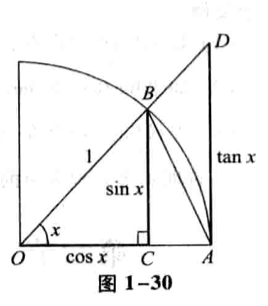## 高等数学记 📖 极限存在准则## 夹逼准则应用例题:

### 1.$\lim_{x\to 0}x\cdot [\frac{10}{x}]$

#### 解:

$x\to 0^+$

$lim_{x\to 0}x\cdot (\frac{10}{x}-1)

$lim_{x\to 0^+}x\cdot \frac{10}{x}-x=10-x=10$

$lim_{x\to 0^+}x\cdot \frac{10}{x}=10$

$x\to 0^-$

$lim_{x\to 0}x\cdot (\frac{10}{x}-1)>lim_{x\to 0}x\cdot [\frac{10}{x}]>\lim_{x\to 0}x\cdot \frac{10}{x}$